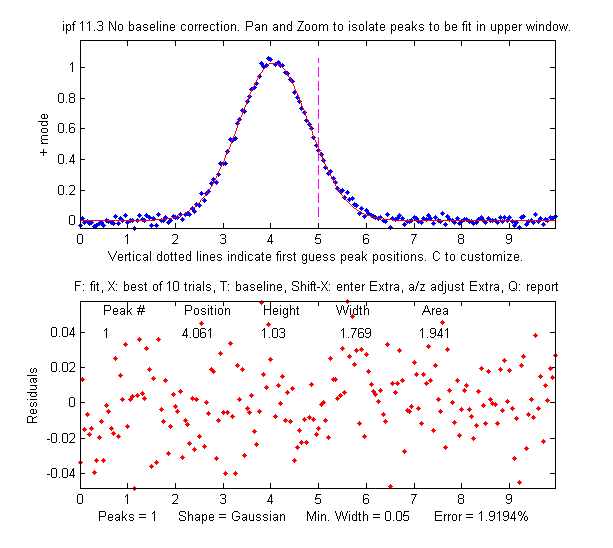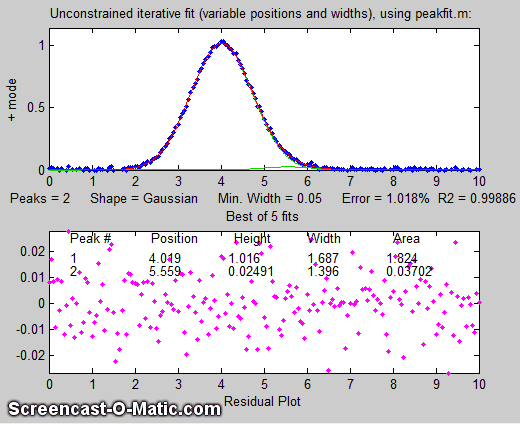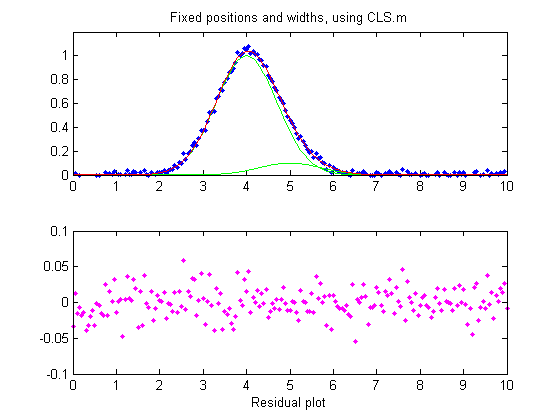[Introduction]  [Signal arithmetic]  [Signals and noise]   [Smoothing]   [Differentiation]  [Peak Sharpening]  [Harmonic analysis]   [Fourier convolution]  [Fourier deconvolution]  [Fourier filter]  [Wavelets]   [Peak area measurement]  [Linear Least Squares]  [Multicomponent Spectroscopy]  [Iterative Curve Fitting]  [Hyperlinear quantitative absorption spectrophotometry] [Appendix and Case Studies]  [Peak Finding and Measurement]  [iPeak]   [iSignal]  [Peak Fitters]   [iFilter]  [iPower]  [List of downloadable software]  [Interactive tools]

### Appendix Q: Measuring a buried peak

This simulation explores the problem of measuring the height of a small peak (also called a "child peak") that is buried in the tail of a much stronger overlapping peak (a "parent peak"), in the especially challenging case that the smaller peak is not even visible to the unaided eye. Three different measurement tools will be explored: iterative least-squares, classical least-squares regression, and peak detection, using the Matlab/Octave tools peakfit.m, cls.m, or findpeaksG.m, respectively. (Alternatively, you could use the corresponding spreadsheet templates).

In this example the larger peak is located at x=4 and has a height of 1.0 and a width of 1.66; the smaller measured peak is located at x=5 and has a height of 0.1 and also with a width of 1.66. Of course, for the purposes of this simulation, we pretend that we don't necessarily know all of these facts and we will try to find methods that will extract such information as possible from the data, even if the signal is noisy. The measured peak is small enough and close enough to the stronger overlapping peak (separated by less than the width of the peaks) that it
never forms a maximum in the total signal. Soit looks like there is only one peak, as shown on the figure on the right. For that reason the findpeaks.m function (which automatically finds peak maxima) will not be useful by itself to locate the smaller peak. Simpler methods for detecting the second peak also fail to provide a way to measure the smaller second peak, such as inspecting the derivatives of the signal (the smoothed fourth derivative shows some evidence of asymmetry, but that could just be due to the shape of the larger peak), or Fourier self-deconvolution to narrow the peaks so they are distinguishable, but that is unlikely to be successful with this much noise. Least-squares methods work better when the signal-to-noise ratio is poor, and they can be fine-tuned to make use of available information or constraints, as will be demonstrated below.

The selection of the best method will depend on what is known about the signal and the constraints that can be imposed; this will depend in your knowledge of your experimental signal. In this simulation (performed by the Matlab/Octave script SmallPeak.m), the signal is composed of two Gaussian peaks (although that can be changed if desired in line 26). The first question is: are there more than one peak there? If we perform an unconstrained iterative fit of a
single Gaussian to the data, as shown in the figure on the right, it shows little or no evidence of a second peak - the residuals look pretty random. (If you could reduce the noise, or ensemble-average even as few as 10 repeat signals, then the noise would be low enough to see graphical evidence of a second peak). But as it is, there is nothing that suggests a second peak.

But suppose we suspect that there
should be another peak of the same Gaussian shape just on the right side of thelarger peak. We can try fitting a pair of Gaussians to the data (figure on the left), but in this case the random noise is enough that the fit is not stable. When you run SmallPeak.m, the script performs 20 repeat fits (NumSignals in line 20) with the same underlying peaks but with 20 different random noise samples, revealing the stability (or instability) of each measurement method. The fitted peaks in Figure window 1 bounce around all over the place as the script runs, as you can see in the animation on the left. The fitting error is on average lower that the single-Gaussian fit, but that by itself does not mean that the peak parameters so measured will be reliable; it could just be "fitting the noise". If it were isolated all by itself, the small peak would have a S/N ratio of about 5 and it could be measured to a peak height precision of about 3%, but the presence of the larger interfering peak makes the measurement much more difficult. (Hint: After running SmallPeak.m the first time, spread out all the figure windows so they can all be seen separately and don't overlap. That way you can compared the stability of the different methods more easily.)But suppose that we have reason to expect that the
two peaks will have the same width, but we don't know what that width might be. We could try an equal width Gaussian fit (peak shape #6, shown in Matlab/Octave Figure window 2); the resulting fit is much more stable and shows that a small peak is located at about x=5 on the right of the bigger peak, shown below on the left.  On the other hand, if we know the peak positions beforehand, but not the widths, we can use a fixed-position Gaussian fit (shape #16) shown on the right  (Figure window 3). In the very common situation where the objective is to measure an unknown concentration of a known component, then it's possible to prepare standard samples where the concentration of the sought component is high enough for its position or width to be determined with certainty.

So far all of these examples have used iterative peak fitting with at least one peak parameter (position and/or width) unknown anddetermined by measurement. If, on the other hand, all the peak parameters are known except the peak height, then the faster and more direct classical least-squares regression (CLS) can be employed (Figure window 4). In this case you need to know the peak position and width of both the measured and the larger interfering peaks (the computer will calculate their heights). If the positions and the heights really are constant and known, then this method gives the best stability and  precision of measurement. It's also computationally faster, which might be important if you have lots of data to process automatically.

The problem with CLS is that it fails to give accurate measurements if the peak position and/or width changes without warning, whereas two of the iterative methods (unconstrained Gaussian and equal-width Gaussian fits) can adapt to such changes. It some experiments it quite common to have small unexpected shifts in the peak position, especially in chromatography or other flow-based measurements, caused by unexpected changes in temperature, pressure, flow rate or other instrumental factors. In SmallPeaks.m, such x-axis shifts can be simulated using the variable "xshift" in line 18. It's initially zero, but if you set it to something greater (e.g. 0.2) you'll find that the equal-width Gaussian fit (Figure window 2) works better because it can keep up with the changes in x-axis shifts.

But with a greater x-axis shift (xshift=1.0) even the equal-width fit has trouble. Still, if we know the separation between the two peaks, it's possible to use the findpeaksG function to search for and locate the larger peak and to calculate the position of the smaller one. Then the CLS method, with the peak positions so determined for each separate signal, shown in Figure window 5 and labeled "findpeaksP" in the table below, works better. Alternatively, another way to use the findpeaks results is a variation of the equal-width iterative fitting method in which the first guess peak positions (line 82) are derived from the findpeaks results, shown in Figure window 6 and labeled "findpeaksP2" in the table below; that method does not depend on accurate knowledge of the peak widths, only their equality.

Each time you run SmallPeaks.m, all of these methods are computed "NumSignals" times (set in line 20) and compared in a table giving the average peak height accuracy of all the repeat runs:

xshift=0
Unconstr. EqualW  FixedP FixedP&W findpeaksP findpeaksP2
35.607    16.849  5.1375  4.4437   13.384     16.849

xshift=1
Unconstr. EqualW  FixedP FixedP&W findpeaksP findpeaksP2
31.263    44.107  22.794  46.18    10.607     10.808

Bottom line. The more you know about your signals, the better you can measure them. A stable signal with known peak positions and widths is the most precisely measurable in the presence of random noise ("FixedP&W"), but if the positions or widths vary from measurement to measurement, different methods must be used, and precision is degraded because more of the available information is used to account for changes other than the ones you want to measure.

This page is part of "A Pragmatic Introduction to Signal Processing", created and maintained by Prof. Tom O'Haver , Department of Chemistry and Biochemistry, The University of Maryland at College Park. Comments, suggestions and questions should be directed to Prof. O'Haver at toh@umd.edu. Updated July, 2022.